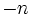# Group of integers

(Redirected from Infinite cyclic group)
View a complete list of particular groups (this is a very huge list!)[SHOW MORE]

## Definition

### Usual definition

The group of integers, typically denoted$\mathbb{Z}$, is defined as follows:

• The underlying set is the set of all integers
• The group operation is integer addition
• The identity element is the integer$0$
• The inverse map is the additive inverse, sending an integer$n$ to the integer$-n$

In the 4-tuple notation, the group of integers in the group$(\mathbb{Z},+, 0, -)$.

### Other definitions

Some other equivalent formulations of the group of integers:

• It is the additive group of the ring of integers
• It is the infinite cyclic group
• It is the free group on one generator
• It is the free abelian group on one generator

## Arithmetic functions

Function Value Explanation
order ((number of elements, equivalently, cardinality or size of underlying set) Infinite (countable) Not a finite group.
exponent Infinite Not a periodic group.
derived length 1 The group is an abelian group.
nilpotency class 1 The group is an abelian group.
Fitting length 1 The group is an abelian group.
Frattini length 1 The group is a Frattini-free group.
subgroup rank of a group 1 The group is cyclic, hence so is every subgroup.

## Group properties

Property Satisfied Explanation Comment
cyclic group Yes
abelian group Yes Cyclic implies abelian
finite group No
finitely generated group Yes Generating set of size one.
slender group Yes Every subgroup is cyclic.
Hopfian group Yes Not isomorphic to any proper quotient, which is finite.
co-Hopfian group No Isomorphic to the proper subgroup generated by any element not the generator or the identity.

## GAP implementation

The group can be defined using the FreeGroup function:

FreeGroup(1)